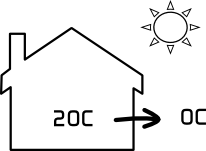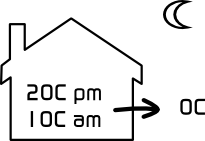# Proof that Heating 24/7 can be Cheaper

This proof is based on a simple thought experiment. Let's take a one room house, 20C inside, 0C outside and loss = 1kW.Day = 16hrs

Loss = 16h x 1kW
Loss = 16kWhNight = 8hrs

Loss = 8h x 1kW
Loss = 8kWh

24hr Loss = 16kWh + 8kWh
24hr Loss = 24kWh

Which is pretty obvious really.

### Case 2. 16/7 Heating

In this situation I have added another assumption which is that the inside temperature falls to 10C overnight while the heating is off. This means the loss is 1kW when the heating goes off but has fallen to 0.5kW 8hrs later, since loss is proportional to the difference between inside and outside temperatures. To keep the maths easy I've taken this as a straight line fall (which gives ~10% error in the night total). Hence the average loss overnight is 0.75kW.Day = 16hrs

Loss = 16h x 1kW
Loss = 16kWhNight = 8hrs

Loss = 8h x 0.75kW
Loss = 6kWh

24hr Loss = 16kWh + 6kWh
24hr Loss = 22kWh

This leads to some interesting conclusions:

• The saving for 8hrs off is ~8% (22/24) which is less that you might expect. It is also 8% for a fall to 10C overnight. If the figures are reworked for a fall to a more reasonable 15C then the saving is only 4%. Hence the better insulated a house is the less you save by turning the heating off.

• The overnight loss comes from the house structure. Most heat loss calculation assume 1 air change per hour so the heat energy is not coming from the air in the house. It must be coming from the structure. This energy must be replaced the next day so during the 16hrs on the central heating has to supply 1.375kW (22kWh/16hr) compared to 1kW for the 24/7 case, an increase of 37.5%. Hence the less the heating is on the higher power it has to deliver.

Note that percentage night saving is independent of the total power, i.e. the size of the house. It just depends on how much the inside temperature falls at night, when the heating is off, relative to the outside temperature.

Similarly the extra output required from the central heating depends mostly on the ratio of on/off. Another way to work this is out is (24hr/on-hrs) x (100%-saving). From which is it clear that the first term is dominant as the second is 92% and upwards (i.e. ~1).

## How this affects costs

So to summarise, turning off the central heating reduces heat loss, but not by much and it also raises the power the central heating has to deliver when it is on. To understand how this can affect costs we have to look indepth at how a typical radiator central heating system functions.

A condensing boiler needs the return water to be at about 55C for condensing to occur. As the typical design drop is 10C that means the outgoing water is 65C and the average radiator temperature is 60C. The radiator temperature for its specified wattage rating is 60C delta, i.e. 80C for a 20C ambient. With a 40C delta (60C radiator, 20C ambient) the output is 60% of the rated figure.

So the shorter the central heating runs the more power it needs to supply when it is on, but condensing mode means low output from the radiators. These are mutally exclusive. If we now factor in the efficiency difference between condensing and non-condensing mode of ~10% then it becomes clear that the choices are:

1. Run longer in condensing mode gaining ~10% in efficiency.
2. Run shorter in non-condensing mode saving 4-8% heat loss.

Maximising condensing mode saves more fuel than shorter running time.

To draw an analogy the energy usage in a car journey is lowest at the lowest speed because both rolling resistance and air resistance increase with speed. However, maximum mpg occurs at ~55mph therefore the cheapest journey cost is a steady 55mph which is not the same as the lowest energy usage.

## Central Heating Rules

For minimum fuel costs I would suggest the following rules:

1. Set the pump as slow as possible to get the maximum drop. If you can get 20C drop then for 55C return the output can be 75C. This helps heating the hot water cylinder quickly as you now have a larger difference. Radiator average temperature is 65C giving 70% of rated figure.
2. Set the output water temperature for 55C return water.
3. Set the heating hours as short as possible. As the weather gets colder, increase the heating hours, not the water temperature. And only as a last resort increase the output water temperature.

Note the above assumes that the boiler is a modulating type where lowering the output water temperature does not affect efficiency. It's impossible to be definitive for on/off boilers where a lower water temperature can cause short-cycling which is inefficient.

## Non-Condensing Boilers

The above of course only applies to condensing boilers which have the option of 2 modes of operation. Non condensing boilers run slightly more efficient with lower water temperature but nothing significant so for them the shortest hours is the cheapest. Non condensing boilers should never be run too cool so that condensing occurs as this will quickly destroy the boiler.